Courses

# Test: Statistics- 1

## 15 Questions MCQ Test Quantitative Aptitude for GMAT | Test: Statistics- 1

Description
This mock test of Test: Statistics- 1 for GMAT helps you for every GMAT entrance exam. This contains 15 Multiple Choice Questions for GMAT Test: Statistics- 1 (mcq) to study with solutions a complete question bank. The solved questions answers in this Test: Statistics- 1 quiz give you a good mix of easy questions and tough questions. GMAT students definitely take this Test: Statistics- 1 exercise for a better result in the exam. You can find other Test: Statistics- 1 extra questions, long questions & short questions for GMAT on EduRev as well by searching above.
QUESTION: 1

### A set X: {a1, a2, a3, a4, a5} with non-zero elements have mean M. What is the mean of the set Y: {a1 - 1, a2 - 2, a3 - 3, a4 + 5, a5 + 1}?

Solution:

In Set X: {a1, a2, a3, a4, a5}

Mean of Set X with 5 elements = M

Sum = Mean x Number of elements
⇒ a1 + a+ a+ a+ a= 5M .. (1)

In Set Y: {a− 1, a− 2, a− 3, a+ 5, a+ 1}

Sum of elements = (a1–1) + (a2–2) +(a3–3) + (a4+5) + (a5+1)

Rearranging, we get,

Sum =a+ a+ a+ a+ a+ (−1 ) + (−2) + (−3) + 5 + 1

Sum  = a+ a+ a+ a+ a+ 0

Sum = 5M  .. From(1)

Mean= (Sum) / (Number of elements)

Mean = 5M/5

Mean =M

QUESTION: 2

### If scores of 10 students in a class are arranged in the table (shown below), then what is the Median of their scores?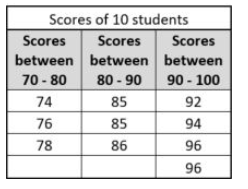Solution:

Score of 10 students in ascending  order = 74, 76, 78, 85, 85, 86, 92, 94, 96, 96

Since number of observations is even, the median is given by finding the average or mean of the two middle most observations.

So, median = (85 + 86)/2

Median = 85.5

QUESTION: 3

### If scores of 10 students in a class are arranged in the table (shown below), then what is the Range of their scores?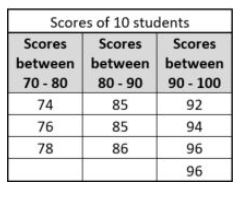Solution:

The difference between the highest and lowest observations in a given data is called its Range.

Score of 10 students in ascending  order = 74, 76, 78, 85, 85, 86, 92, 94, 96, 96

Then the difference between the highest and lowest value will be 96 - 74

Range = 22

QUESTION: 4

Set X is given by {a, 2a, 3a, 4a, 5a} where ‘a’ is a positive integer. If element ‘a’ in Set X is replaced by ‘b’ and b < a, then which of the following must be true?

I.Mean would not change.

II.Median would not change.

III. Standard deviation would not change

Solution:
QUESTION: 5

Set A: {-11, 0, 0, 11}

Set B: {2, 4, 6, 8}

Set C: {50, 51, 52, 53}

Set D: {12, 12, 12, 12}

Which of the following sets have a maximum & minimum standard deviation respectively?

Solution:
QUESTION: 6

If the medians of the List A and List B are same, what is the value of k?

List A: 2, 5, 7, 20

List B: 2, 5, 7, 20, k

Solution:

Step 1: Question statement and Inferences

We need to find the median of List B and then compare the value of k

Step 2: Finding required values

Median of List A = (5+7)/2 = 6

Given: Median of List A = Median of List B

Therefore, Median of List B = 6

Step 3: Calculating the final answer

As there are five elements in List B, the median will be the middle number

As no element is equal to 6, k must be 6.

(Observe that 6 is exactly the middle number of List B).

QUESTION: 7

What is x, if the average of five numbers, x, 6, 3, 15 and 12 is equal to the median?

(1)  6 < x < 12

(2)  x is median of the five numbers

Solution:

Steps 1 & 2: Understand Question and Draw Inferences

Arithmetic mean is same as median

• As there are five elements, median must be one of them

Step 3: Analyze Statement 1

6<x<12

• As x is less than two numbers (12, 15) and greater than the other two (3, 6), it must be the median
• x is the average of all the numbers

It is given that the mean is same as the median

• (3+6+12+15+x)/5 = x
• 3+6+12+15+x = 5x
• 4x = 36
• x = 9

Therefore statement 1 is sufficient.

Step 4: Analyze Statement 2

x is median of the five numbers

It is given that the mean is same as the median

• (3+6+12+15+x)/5 = x
• 3+6+12+15+x = 5x
• 4x = 36
• x = 9

Therefore statement 2 is sufficient.

Step 5: Analyze Both Statements Together (if needed)

We get a unique answer in step 3 and step 4, so this step is not required

QUESTION: 8

A, B, C, D, E, and F are six consecutive positive odd integers in increasing order. What is the value of the median of these six integers?

(1) The sum of the two smallest integers is greater than the largest integer by 13

(2) The average (arithmetic mean) of these integers is 26

Solution:

Steps 1 & 2: Understand Question and Draw Inferences

We are given six consecutive positive odd integers. We have to find the median of this set of integers.

Since the integers are consecutive odd integers, they can be represented with the help of a positive integer n, as follows:

A = 2*n + 1

B = 2*n + 3

C = 2*n + 5

D = 2*n + 7

E = 2*n + 9

F = 2*n + 11

Now, we know that, for a series in increasing order, the median is the middle number in case of odd number of integers and the average of the middle two numbers in case of even number of integers.

Since there are even number (6) of integers in this series, the median will be the average of the 3rd and 4th number: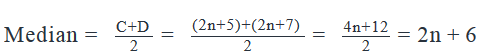Thus, in order to find the value of the median, we need to know the value of n. So, the question becomes “what is the value of n?”

Step 3: Analyze Statement 1

The sum of the two smallest integers is greater than the largest integer by 13 ?

Now, the two smallest integers in the sequence are 2n+1 and 2n+3 and their sum = 4n+4

The largest integer in the sequence is 2n+11.

Per statement (1), the difference between these two should be 13. So,

(4n+4) – (2n+11) = 13

2n-7 = 13

2n = 20

Thus, n =10.

Now, since we have found the value of n, we can find the median.

Hence, statement (1) is sufficient to find a unique value of the median.

Step 4: Analyze Statement 2

The average (arithmetic mean) of these integers is 26

Thus: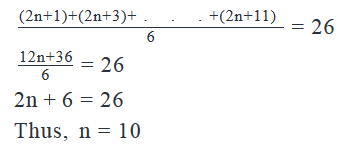Now, since we have found the value of n, we can find the median.

Hence, statement (2) is sufficient to find a unique value of the median

Step 5: Analyze Both Statements Together (if needed)

Since statement (1) and (2) alone are sufficient to answer the question, we don’t need to perform this step.

QUESTION: 9

10, 6, p, q, 11

Given the above series of positive integers arranged in a random order, what is the value of p/q?

(1)  The average (arithmetic mean) of given numbers is 7

(2)  p is the median of the given series

Solution:

Steps 1 & 2: Understand Question and Draw Inferences

We are given that a series consist of 5 elements arranged in a random order. The numbers are 10, 6, p, q, and 11 where p and q are positive integers.

We have to find the value p/q. For that, we need to find the value of p and q.

Since there is no other information provided in the question, let’s move on to the analysis of statements 1 and 2.

Step 3: Analyze Statement 1

The average (arithmetic mean) of given numbers is 7

Now, the average of these numbers can be calculated as follows: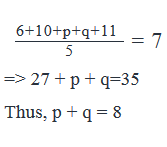However, there are multiple options available for the values of p and q:

p = 7, q = 1 OR p = 1, q = 7

p = 6, q = 2 OR p = 2, q = 6

p = 5, q = 3 OR p = 3, q = 5

p = 4, q=4

Hence, statement 1 alone is not sufficient to answer the question.

Step 4: Analyze Statement 2

p is the median of the given series

The fact that p is the median of the series tells us only that if the numbers are arranged in ascending or descending order, the number p will come at the third place.

The following table lists all the possible arrangements and the corresponding values of (p,q) that are possible: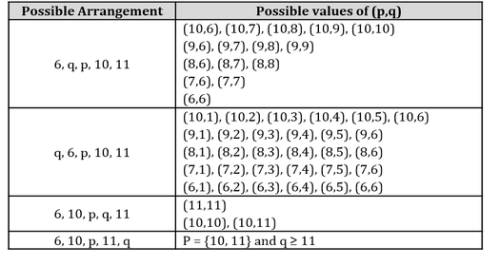Thus, we see that there exist multiple sets of possible values of (p,q)

Hence, statement 2 alone is not sufficient to answer the question.

Step 5: Analyze Both Statements Together (if needed)

From Statement 1,

p + q = 8

From Statement 2,

All the sets of (p,q) in the table above are possible

By combining both sets,

Let’s pick those sets from the table above for which p + q = 8

Such sets of (p, q) are:

(7,1), (6,2)

For the set (7,1), the value of p/q is 7

For the set (6,2), the value of p/q is 3

Thus, we don’t get a unique value of the ratio p/q.

Both statements combined are not sufficient to answer the question.

QUESTION: 10

From January to October, the revenue of a textile manufacturing plant averaged 20,000 USD per month. What average monthly revenue in USD was required in November and December, if the plant had a goal to achieve 330,000 USD in revenue by the end of the calendar year?

Solution:

Step 1: Question statement and Inferences

We are given that the average revenue for a textile manufacturing plant was 20,000 USD per month from January till October. The plant wanted to achieve a total revenue of 330,000 USD by the end of the year.

We have to find the average revenue that the plant must achieve in November and December to meet its total targeted revenue.

Let’s say the monthly revenues for the plant are M1, M2, M3 ….. M12.

Now, we don’t know the individual values of the monthly revenues. But we do know that the average monthly revenue for the first ten months is USD 20,000. Thus,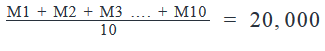Hence,

M1 + M2 + M3 …. + M10 = 200,000   ………… (1)

The targeted revenue for the year is USD 330,000. Thus,

M1 + M2 + M3 …. + M11 + M12 = 330,000 ………… (2)

Step 2: Finding required values

The question wants us to find the average monthly revenue for November and December. Let’s say the average monthly revenue for these two months is X. Thus,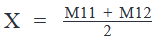That is, M11 + M12 = 2X      ………… (3)

Substituting values from Equations 1 and 3 in Equation 2:

200,000 + 2X = 330,000

2X = 130,000

X = 65,000

So, the average monthly revenue for the months of November and December is 65,000 USD.

QUESTION: 11

What is the mean of an increasing sequence of 10 consecutive integers?

(1)  The mean of the first 7 integers is 7.

(2)  The mean of  the last 7 integers is 10.

Solution:

Step 1 & 2 – Understand the question and draw inferences from the question statement.

We have to find the mean of an increasing sequence of 10 consecutive integers

Let the integers be a, a+ 1, a+ 2, a+ 3, a+ 4, a+ 5, a+ 6, a+ 7, a+ 8 and a+ 9, where a is the lowest integer:

Sum of the 10 consecutive integers

= a+ a+ 1 + a+ 2 + a+ 3 +a+ 4 +a+ 5 + a+ 6 +a+ 7 +a+ 8 +a+ 9

= 10a + 45

Mean of {a1, …..a1 + 9} = (10a+45)/10

If we can find the value of a, we can find the mean.

Step 3 – Analyze Statement 1 Independently

Statement 1 – The mean of the first 7 integers is 7

We Inferred: first seven integers are: a, a+ 1, a+ 2, a+ 3, a+ 4, a+ 5, a+ 6

Their Sum

= a+ a+ 1 + a+ 2 + a+ 3 +a+ 4 +a+ 5 + a+ 6

= 7a + 21

Mean of {a1, ….., a1 + 7} =  (7a +21)/7 = a + 3

Given Mean = 7

• a + 3 = 7
• a = 7 -3 = 4

Statement 1 is independently sufficient to arrive at a unique answer.

Step 4 – Analyze Statement 2 Independently

Statement 2 -The mean of the last 7 integers is 10.

We Inferred: last seven integers are: a+ 3, a+ 4, a+ 5, a+ 6, a+ 7, a+ 8, a+ 9

Their Sum

= a+ 3 + a+ 4 + a+ 5 +a+ 6 +a+ 7 + a+ 8 +a+ 9

= 7a + 42

Mean of {a1,….., a1 + 7} = (7a +42)/7 = a + 6

Given Mean = 10

• a + 6 = 10
• a = 10 -6 = 4

Statement 2 is independently sufficient to arrive at a unique answer.

Step 5 – Analyze Both Statements Together

We get a unique value in Step 3 and Step 4

Statement (1) and (2) are independently sufficient to arrive at a unique answer.

QUESTION: 12

What is the standard deviation of {-3, -6, -5, -10} approximately equal to, if the standard deviation of {3, 6, 5, 10} is approximately equal to 2.5?

Solution:

Finding standard deviation of {-3, -6, -5, -10}

Step 1

Mean of {-3,-6,-5,-10} = {( -3) + (-6) + (-5) + (-10)}/4 = -6

Step 2

Distances of each point from the mean:

• -3 – (-6) = 3
• -6 – (-6) = 0
• -5 – (-6) = 1
• -10-(-6) = -4

Step 3

Squared Distance from Mean

• = (3)2 + (0)2 + (1)2 + (-4)2
• = 9 + 0 + 1 + 16 = 26

Step 4

• Average = 26/4 = 6.5

Step 5

• Standard Deviation = √6.5 ≌ 2.5
• Note that this Standard Deviation is the same as Standard deviation of {3, 6, 5, 10}.

Remember -The standard deviation of a set remains the same even if all the elements are multiplied by (-1)

QUESTION: 13

Some milk was added to each of the 5 cans. If standard deviation of the quantity of milk in the 5 cans at the beginning was 3.5 liters, what was the standard deviation of the quantity of milk in the 5 cans at the end?

(1)  In each can, 20% of existing amount of milk was added.

(2)  The average volume of milk in the cans at the end was 25 liters.

Solution:

Step 1 & 2 – Understand the question and draw inferences from the question statement.

To Find:  We have to find the standard deviation of the volume of milk in 5 cans after some was added to each of the cans.

Given: Standard Deviation of the 5 cans of milk in the beginning is 3.5 liters.

Let the quantity of milk in 5 cans be a, b, c, d and e.

Finding standard deviation of {a, b, c, d, e}

Step 1

Mean of {a,b,c, d, e} = {a + b + c + d + e}/5 = M

Step 2

Distances of each point from the mean:

• a-M
• b-M
• c-M
• d-M
• e-M

Step 3

Squared Distance from Mean

• = (a -M)2 + (b-M)2 + (c -M)2 + (d -M)2 + (e -M)2

Step 4

Average = {(a -M)2 + (b-M)2 + (c -M)2 + (d -M)2 + (e -M)2}  /5

Step 5

• Standard Deviation = √ [{(a -M)2 + (b-M)2 + (c -M)2 + (d -M)2 + (e -M)2}  / 5] = 3.5

Step 3 – Analyze Statement 1 Independently

Statement 1 – In each can, 20% of the existing quantity of milk was added

The amount of milk be a+20%a, b+20%b, c+20%c, d+20%d, e+20%e

Remember:  If all the elements of a set are increased by the x%, the standard deviation of the set also increases by the x%.

So the standard deviation of this set will increase by 20%

New S = 3.5 + 20% *3.5 = Unique number

Alternatively you can calculate the standard deviation of this set as shown below by following same step by step method.

Set = { a+20%a, b+20%b, c+20%c, d+20%d, e+20%e }

Step 1

• Sum of {a+20%a, b+20%b, c+20%c, d+20%d, e+20%e}
• = (1.2)(a + b + c + d + e)
• Mean of {a+20%a, b+20%b, c+20%c, d+20%d, e+20%e}
• = (1.2)(a + b + c + d + e)/5 = 1.2 M

Step 2

Distances of each point from the mean:

• 1.2 (a –M)
• 1.2 (b-M)
• 1.2(c-M)
• 1.2(d-M)
• 1.2(e-M)

Step 3

Squared Distance from Mean

• = 1.22 [(a -M)2 + (b-M)2 + (c -M)2 + (d -M)2 + (e -M)2 ]

Step 4

• Average = 1.22{(a -M)2 + (b-M)2 + (c -M)2 + (d -M)2 + (e -M)2}  /5

Step 5

• Standard Deviation = 1.2 √ [{(a -M)2 + (b-M)2 + (c -M)2 + (d -M)2 + (e -M)2}  / 5]
• = 1.2 *3.5 = 3.5 + 20% *3.5
• = Unique value

Statement (1) is independently sufficient to arrive at a unique answer.

Step 4 – Analyze Statement 2 Independently

Statement 2 -The average quantity of milk in the cans at the end was 25 liters

We do not know how the quantity changed in each of the cans.

Thus, the information given in statement (2) is not sufficient to arrive at a unique solution.

Step 5 – Analyze Both Statements Together

We get a unique value in Step 3.

Statement (1) independently is sufficient to arrive at a unique answer

QUESTION: 14

Set X consists of 10 integers and has median of 20 and a range of 20. What is the value of the greatest possible integer that can be present in the set?

Solution:

Given:  Median and Range

To Find: The greatest possible number

Let the lowest number be P and the greatest number be Q

Given:

• Range = 20
• Q – P = 20
• Q = 20 + P

Here we expressed Q (the highest value) in terms of P (the lowest value).

Therefore by finding the max value of P, we can find the max value of Q.

Now we know that the median = 20

• So, the greatest value which P can take = 20
• This means that at least 6 numbers in the given set of 10 numbers will have the same value of 20. This ways, when the 10 numbers are arranged in an ascending order, the median will be equal to half the sum of the 5th and 6th term. And so, will be equal to 20. The 7th, 8th and 9th terms (when the 10 numbers are arranged in an ascending order) can take any value between 20 and 40. The 10th term will be 40.
• That is, Q = 40
• Thus, the greatest integer that can be present in this set is 40.

Remember -The difference between any two numbers in a set cannot be greater than the range.

QUESTION: 15

S is standard deviation of a, b, and c, if a constant C is added to all the three digits, the new standard deviation will be?

Solution:

Finding standard deviation of {a, b, c}

Step 1

Let Mean of {a,b,c} = {a + b + c}/3 = M

Step 2

Distances of each point from the mean:

• a-M
• b-M
• c-M

Step 3

Squared Distance from Mean

• = (a -M)2 + (b-M)2 + (c -M)2

Step 4

• Average = {(a -M)2 + (b-M)2 + (c -M)2}  /3

Step 5

• Standard Deviation = √ {(a -M)2 + (b-M)2 + (c -M)2}  /√ 3 = S

Finding standard deviation of {a+C, b+C, c+C}

Remember: The standard deviation of a set remains the same even if a constant is added to all the elements of the set

So the standard deviation of this set should be S.

Alternatively you can calculate the standard deviation of this set as shown below by following same step by step method.

Step 1

Mean of {a+C, b+C, c+C}  = (a + b + c )/3 + C = M + C

Step 2

Distances of each point from the mean:

• a+C – M-C = a – M
• b+C -M-C = b-M
• c+C – M-C = c-M

Step 3

Squared Distance from Mean

• = (a -M)2 + (b-M)2 + (c -M)2

Step 4

• Average = {(a -M)2 + (b-M)2 + (c -M)2}  /3

Step 5

Standard Deviation = √ {(a -M)2 + (b-M)2 + (c -M)2}  /√ 3 = S

Track your progress, build streaks, highlight & save important lessons and more!

### Similar Content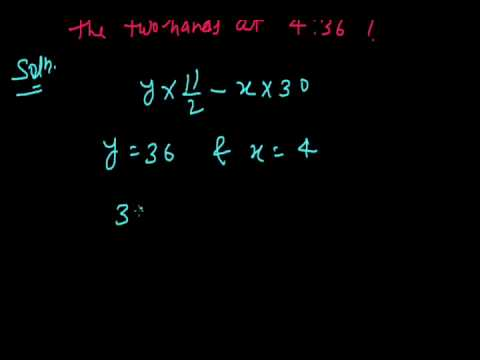### Related tests